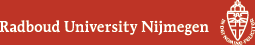## Geometry Seminar - Abstracts

### Talk

Thursday 25 October 2018, 16:00-17:00 in HG03.085
An orbital integral of a function on a group $$G$$ is its integral over a conjugacy class in $$G$$. If such an orbital integral defines a continuous functional on a convolution algebra $$A(G)$$ of functions on $$G$$, then it is a trace on that algebra. If the conjugacy class consists of just the identity element, this is the classical von Neumann trace. In general, such a trace induces a map on the $$K$$-theory of $$A(G)$$with values in the complex numbers. If $$A(G)$$ is dense in the reduced or full group $$C^*$$-algebra of G and closed under holomorphic functional calculus, then this gives a map on the K-theory of that group $$C^*$$-algebra. It has turned out in recent years that such maps are useful tools for studying elements of these $$K$$-theory groups. This is true in particular for $$K$$-theoretic indices of $$G$$-equivariant elliptic operators. Index formulas for the numbers obtained in this way have turned out to have implications to representation theory and geometry. In this talk, I will discuss this development, including joint work with Hang Wang.